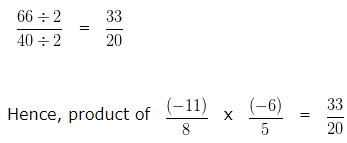# Multiplication of Rational Numbers | Examples | Maths

Multiplication of Rational Numbers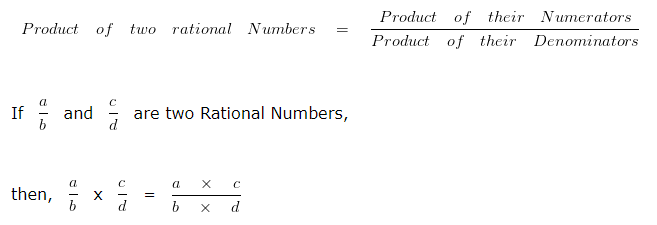### Multiplication of Rational Numbers Examples

Example 1

Find the product of 15/7 and 3/5?

Explanation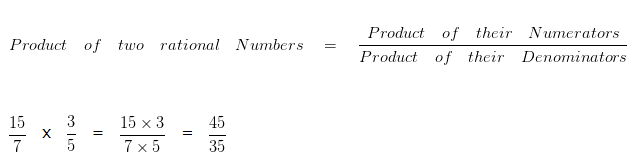To further simplify the given numbers into their lowest form, we would divide both the Numerator and Denominator by their HCF

HCF of 45 and 35 is 5

Dividing both the Numerator and Denominator by their HCF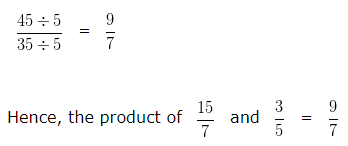Multiplication of Rational Numbers – Example 2

Find the product of 9/7 and -12/8?

Explanation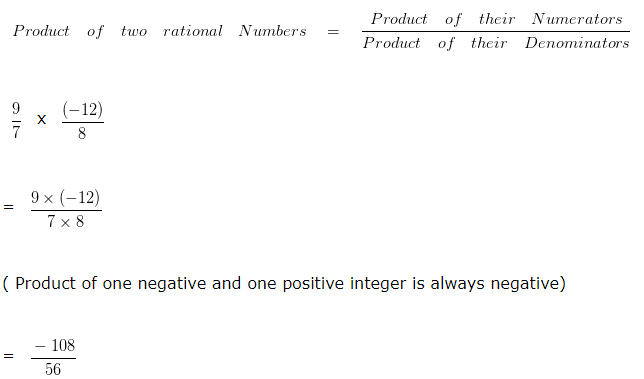To further simplify the given numbers into their lowest form, we would divide both the Numerator and Denominator by their HCF

HCF of 108 and 56 is 4

Dividing both the Numerator and Denominator by their HCF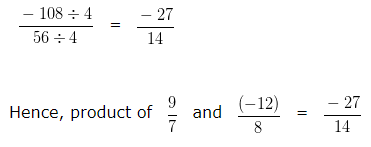Multiplication of Rational Numbers – Example 3

Find the product of -4/7 and 8/12?

Explanation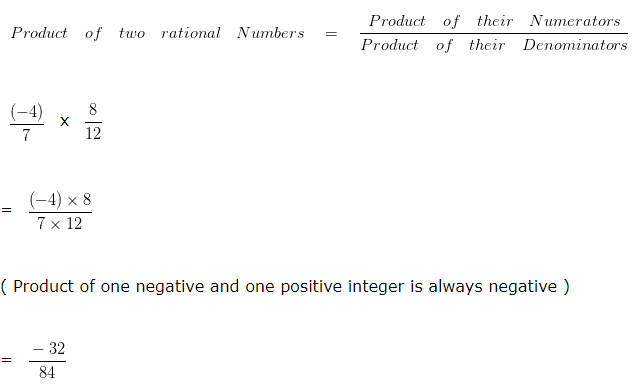To further simplify the given numbers into their lowest form, we would divide both the Numerator and Denominator by their HCF

HCF of 32 and 84 is 4

Dividing both the Numerator and Denominator by their HCF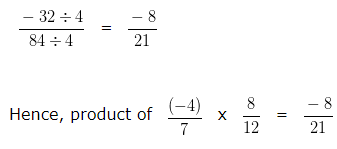Multiplication of Rational Numbers – Example 4

Find the product of -11/8 x -6/5?

Explanation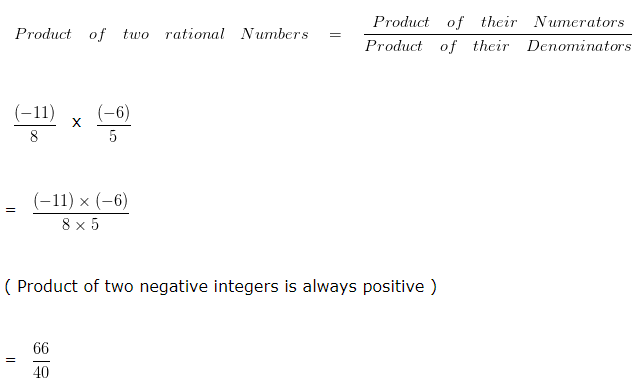To further simplify the given numbers into their lowest form, we would divide both the Numerator and Denominator by their HCF

HCF of 66 and 40 is 2

Dividing both the Numerator and Denominator by their HCF